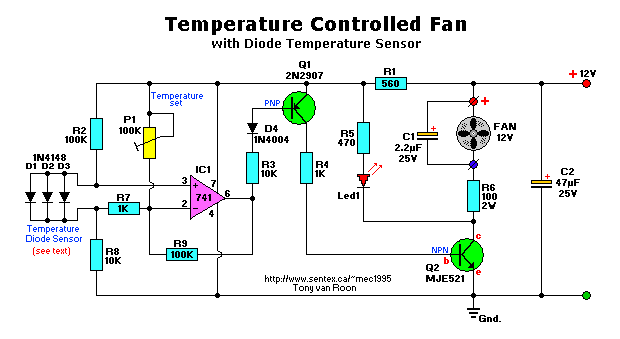```Parts List:
R1 = 560 ohm                                Q1 = 2N2907 (NTE159M) low noise
R2,R9 = 100K                                   Q2 = MJE521 (NTE184)
R3,R8 = 10K                                   IC1 = 741, op-amp
R4,R7 = 1K                                   Led1 = LED, red
R5 = 470 ohm                          D1,D2,D3 = 1N4148, signal diode
R6 = 100 ohm, 2watt, wire-wound             D4 = 1N4004, general purpose diode
P1 = 100K, trimmer pot

C1 = 2.2uF, 25V, electrolytic
C2 =  47uF, 25V, electrolytic
```
This circuit controls very accurately a fan of any size. Just adjust the associated resistors for a different type like the R6 resistor of 100 ohm, 2 watt type and you're all set. The above circuit diagram is for a small 12 volt fan, the size and type determined by the user.

Temperature is sampled via the 1N4148 diodes and presented at pins 2 and 3 of the differential type 741 op-amp. R7 (10K) is used to create a voltage difference between the inverted and non-inverted input pins 2 and 3 of the 741. All signals presented at pin 2 will be inverted on the output pin 6. Obviously then, the input pins are very important. When pin 2 goes more positive than pin 3, the output pin 6 of the 741 goes high and forward biasing the base of transistor Q1, which switches on transistor Q2 and the Led and puts 12V on the output pins for the fan. R9 functions as a feedback for the 741. Only DC type fans can be used with this schematic diagram without further modifications.

The temperature sensor is made up of three easily available 1N4148 signal diodes mounted in parallel. Mount them in a thin aluminum, or plastic tube (depending on your application) and silicon the end of the tube to make this temperature sensor water-proof. As an additional note, I have seen this type of temperature sensor, with the diodes either in parallel or series and either 1 or more diodes, in all sorts of laboratory equipment like hot water baths and others. The water bath temperature setting ranged from room temperature to about 100° Celsius. Keep in mind that using the 1N4148 diode as a temperature sensor is very accurate when used within the specifications of the 1N4148.

Back to Circuit's Page